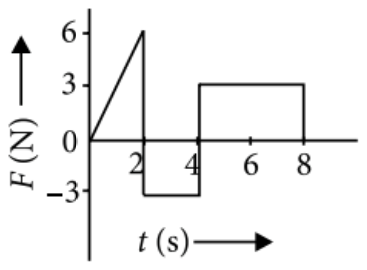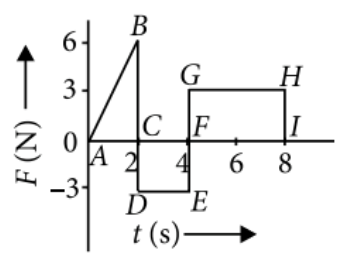Second law
Question

# The force F acting on a particle of mass m is indicated by the force-time graph shown below. The change in momentum of the particle over the time interval from zero to 8s isModerate
Solution

##Change in momentum = Area under F-t graph in that interval = Area of $∆$ABCD - Area of rectangle CDEF + Area of rectangle FGHI

Get Instant Solutions